Function Repository Resource:

# ConsecutiveSquareSum

Find integers representable as the sum of consecutive squares

Contributed by: Jan Mangaldan
 ResourceFunction["ConsecutiveSquareSum"][n] finds integers that can be represented as sums of both n and n+1 consecutive squares. ResourceFunction["ConsecutiveSquareSum"][n,k] finds k such integers.

## Details

ResourceFunction["ConsecutiveSquareSum"][n] returns a tuple {p,q,t}, where t is the sum of squares of n consecutive integers starting from p, and n+1 consecutive integers starting from q.

## Examples

### Basic Examples (2)

Find an integer that can be represented as the sum of two and three consecutive squares:

 In:=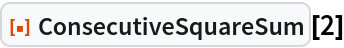Out=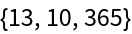Check that 365 satisfies this property:

 In:=Out=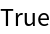Find four integers that can be represented as the sum of two and three consecutive squares:

 In:=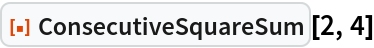Out=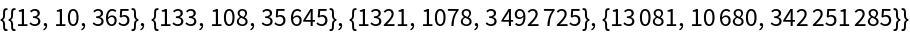Check all the results:

 In:=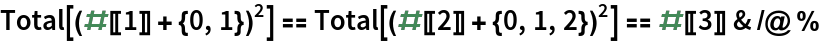Out=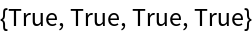### Scope (2)

Find five integers representable as the sum of 100 and 101 squares:

 In:=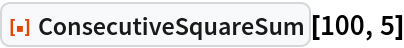Out=Verify the first example:

 In:=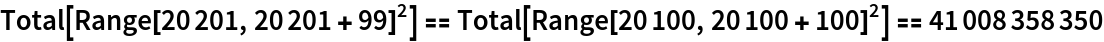Out=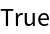## Version History

• 1.0.0 – 10 February 2021